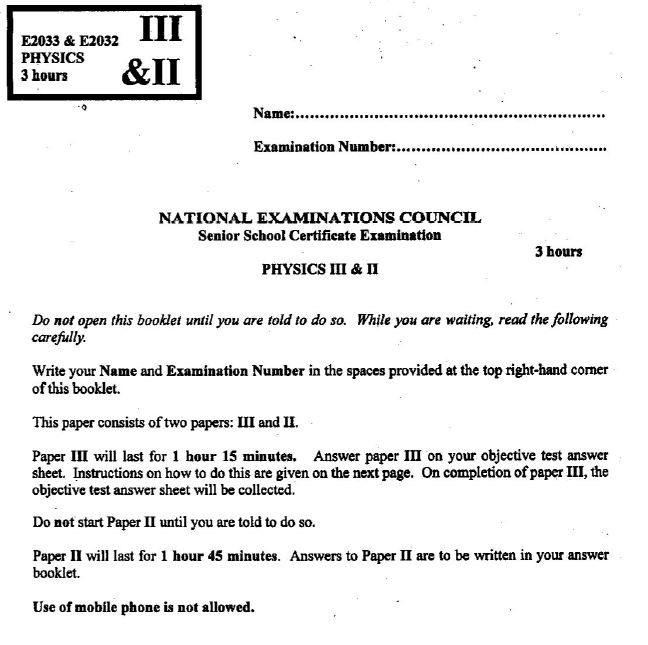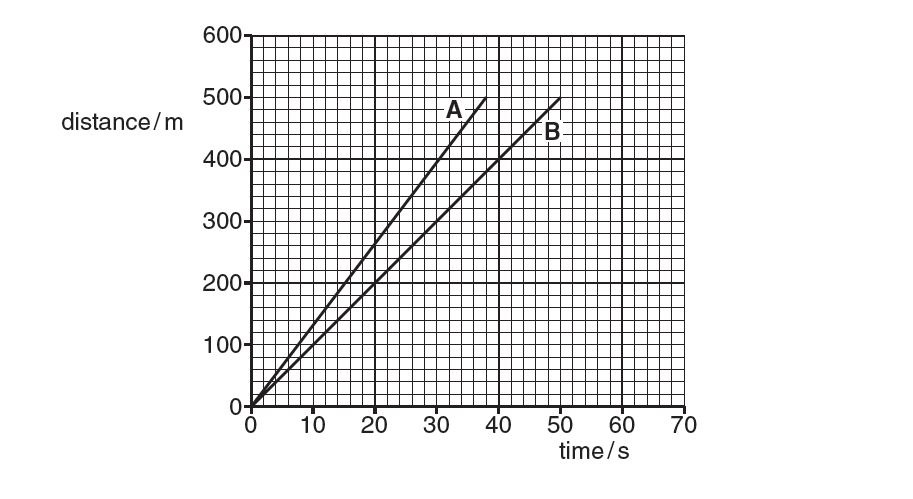# NECO GCE Physics Answers 2018 is Out – See Obj/Essay Questions

## NECO GCE Physics 2018 Answers with Theory and Objective Questions Released.

The Neco Gce Physics answers 2018 is now available. The National Examination Council (NECO) has announced that the General Certificate Examination (GCE) Physics paper will be written today, Tuesday, 27th November, 2018.

The Neco Gce physics exam is for Paper III & II: Objective & Essay which will commence from 2.00pm and end by 5.00pm. That means the examination will last for three hours.

In this post, we will be posting out samples of the neco gce physics questions for candidates that will participate in the examination. Continue reading.Note that below is the questions from Neco past questions and answers that we feel are likely questions for GCE preparation. Keep following this page and make sure you bookmark this site for reference purposes.

### Neco Gce Physics Theory 2018:

1. A man rides a motorcycle 12 km due east and later 5 km due south. Calculate the:
(a) total distance covered;
(b) magnitude of the displacement of the man.

2. Fig. 1.1 shows the distance-time graph for two cyclists A and B. They start a 500 m race together but finish the race at different times.(a) Use Fig. 1.1 to determine
(i) the distance between A and B at time t = 20s,
(ii) the difference in the time taken by A and B for the race.

(b) Cyclist C starts the race at the same time as A and B and covers the first 200m of the race at a constant speed of 5.0 m/s. He then accelerates and finishes the race at t = 60s.
(i) On Fig. 1.1, draw the distance-time graph for cyclist C.
(ii) Calculate the average speed of cyclist C for the whole race.

3. State:
(a) the difference between plane polarized light and ordinary light;
(b) two uses of polaroids.

4. A direct current of 10 A is passed through a voltameter continuously for 1 hour in order to deposit m grammes of a metal of electrochemical equivalent 1.2 x 10-7 kg C-1. Calculate m.

5. (a) (i) What is a ‘lightning conductor’?
(ii) Why are lightning conductors used to protect tall buildings from the undesirable effect of thunderstorms?
(b) Draw the lines of force associated with the following situations:
(i) Two unlike point charges of equal magnitude;
(ii) A positive charge in isolation;
(iii) Two parallel plates carrying unlike charges of equal magnitude.
(c) Two equal point charges exert a force F on each other when they are 5.0 x 10-2m apart in a vacuum. Determine the distance between the charges if the force is to be doubled.

6. Give three observations in support of de-Broglie’s assumption that moving particles behave like waves.

7. Electrons from a hot cathode are accelerated from rest to a target in a vacuum tube maintained at a potential difference of 180V.  Calculate the velocity of the electrons on reaching the target.
[ Mass of an electron = 9.1 x 10-31 kg; charge of an electron = 1.6 x 10-19 C]

8. Explain ‘threshold frequency’ with respect to photoemission.

9.

Note: There is nothing like Neco GCE Physics Expo online. Neco GCE candidates are to desist from patronizing online fraudsters / vendors who says they can provide such services as they are not real.

### Neco Gce Physics Objective:

If a satellite travels at a constant speed round the earth in a circular orbit,

1. Which of the quantities in the list below are scalars.
(a) acceleration (b) force (c) mass (e) speed.

2. The velocity of the satellite changes, but its speed is constant. State what is meant by velocity…………………….

3. Explain why the velocity changes……………………………

4. Explain what makes this satellite move in an orbit that is circular…………………………

5.

If you have any questions, endeavour to use the comment box below…